Thermodynamics and Propulsion
Subsections

# 3.5 The Internal combustion engine (Otto Cycle)

[VW, S & B: 9.13]

The Otto cycle is a set of processes used by spark ignition internal combustion engines (2-stroke or 4-stroke cycles). These engines a) ingest a mixture of fuel and air, b) compress it, c) cause it to react, thus effectively adding heat through converting chemical energy into thermal energy, d) expand the combustion products, and then e) eject the combustion products and replace them with a new charge of fuel and air. The different processes are shown in Figure 3.8:

1. Intake stroke, gasoline vapor and air drawn into engine (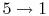).
2. Compression stroke,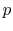,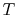increase (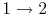).
3. Combustion (spark), short time, essentially constant volume (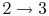). Model: heat absorbed from a series of reservoirs at temperatures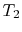to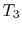.
4. Power stroke: expansion (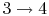).
5. Valve exhaust: valve opens, gas escapes.
6. (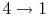) Model: rejection of heat to series of reservoirs at temperatures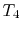to.
7. Exhaust stroke, piston pushes remaining combustion products out of chamber (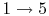).

We model the processes as all acting on a fixed mass of air contained in a piston-cylinder arrangement, as shown in Figure 3.10.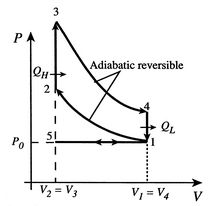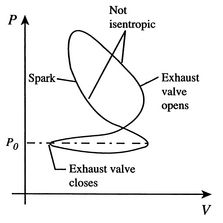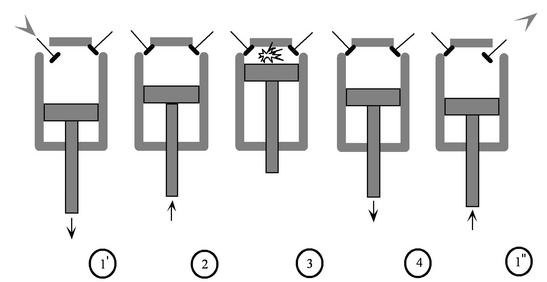The actual cycle does not have the sharp transitions between the different processes that the ideal cycle has, and might be as sketched in Figure 3.9.

## 3.5.1 Efficiency of an ideal Otto cycle

The starting point is the general expression for the thermal efficiency of a cycle: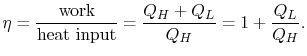The convention, as previously, is that heat exchange is positive if heat is flowing into the system or engine, so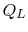is negative. The heat absorbed occurs during combustion when the spark occurs, roughly at constant volume. The heat absorbed can be related to the temperature change from state 2 to state 3 as: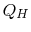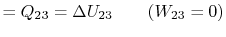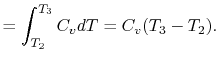The heat rejected is given by (for a perfect gas with constant specific heats)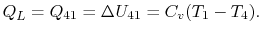Substituting the expressions for the heat absorbed and rejected in the expression for thermal efficiency yields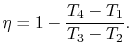We can simplify the above expression using the fact that the processes from 1 to 2 and from 3 to 4 are isentropic: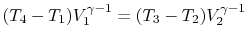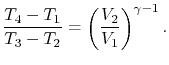The quantity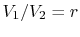is called the compression ratio. In terms of compression ratio, the efficiency of an ideal Otto cycle is: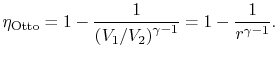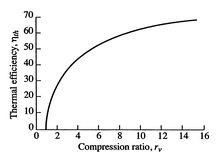The ideal Otto cycle efficiency is shown as a function of the compression ratio in Figure 3.11. As the compression ratio,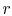, increases,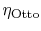increases, but so does. Ifis too high, the mixture will ignite without a spark (at the wrong location in the cycle).

## 3.5.2 Engine work, rate of work per unit enthalpy flux

The non-dimensional ratio of work done (the power) to the enthalpy flux through the engine is given by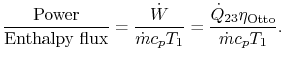There is often a desire to increase this quantity, because it means a smaller engine for the same power. The heat input is given by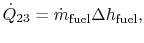where
•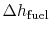is the heat of reaction, i.e. the chemical energy liberated per unit mass of fuel,
•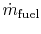is the fuel mass flow rate.
The non-dimensional power is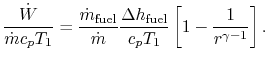The quantities in this equation, evaluated at stoichiometric conditions are: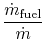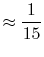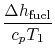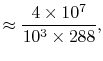so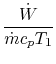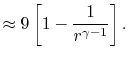Muddy Points

How iscalculated? (MP 3.6)

What are stoichiometric conditions?'' (MP 3.7)

UnifiedTP